### Home > CAAC > Chapter 2 > Lesson 2.1.1 > Problem2-10

2-10.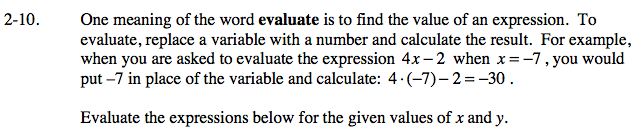Rewrite the expression with the number in place of the variable and simplify if possible.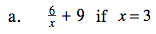$\frac{ 6 }{(3) } + 9 =$

$\text{Remember }\frac{6}{3} \text{ is the same as }6 \text{ divided by }3.$

11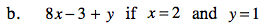8(2) − 3 + (1)

Try evaluating this one on your own. Think of the rules for multiplying positive and negative integers.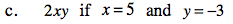2(5)(−3) = 10(−3)

−30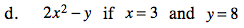2(3)2 − (8)